# How to Calculate the Percentage Based on Different Data Type and Table Structure in Excel

We often do statistics analysis by Excel in our daily work, and sometimes we need to count the percentage for different data in a series of data, so that we can see the data distribution of this series of data and we can also find out which data accounts the largest proportion. Due to different data type and table structures, we will use different methods to calculate the percentage. So, in this article, we will provide you some methods to calculate percentage based on different situations. You can use the formula including COUNTIF and COUNTA functions to calculate percentage, or use SUM function, or insert a PivotTable per your demands. Please continue to read this article, select one proper method to solve your problem.

## Method 1: Count the Percentage by COUNTIF and COUNTA Functions in Excel

See the example below. We do a simple statistic for fruit category on weekdays.

If we want to know which fruit is the most common occurs for a week, you can count the percentage for each of them. Let’s follow below steps to learn how to count the percentage.

Step 1: Create a table like below. D column lists all fruit categories. E column lists the percentage for each fruit.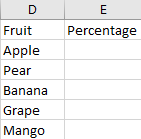Step 2: In E2, enter the formula =COUNTIF(\$B\$2:\$B\$11,D2)/COUNTA(\$B\$2:\$B\$11). In this formula, COUNTIF counts the number for D2 (Apple) in the absolute range \$B\$2:\$B\$11, COUNTA counts all cells number in the absolute range \$B\$2:\$B\$11. Then use COUNTIF divide COUNTA we can get the percentage.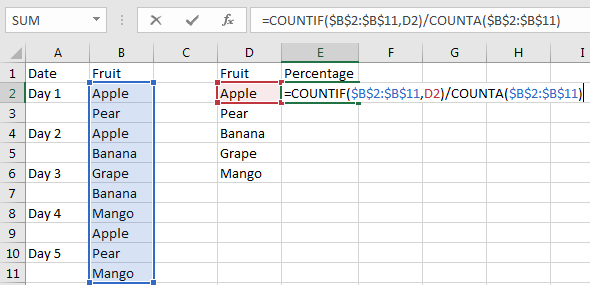Step 3: Click Enter to get the result.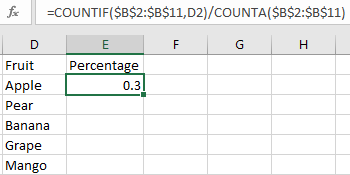Step 4: Drag the fill handle to fill the following cells. Verify that all percentages are displayed.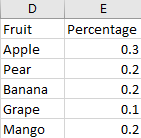Notes:

1. This method is typically used to count the percentage of the times a value appears in a set of data.
2. This method doesn’t take effective if the data is a number. So, we need another method to calculate the percentage for a number among a batch of numbers in Excel.

## Method 2: Calculate the Percentage by SUM Function in Excel

See the example below.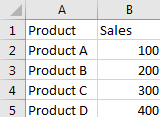If we use above method 1 to calculate percentage of the sales for product A, we will get an improper value. See screenshot below: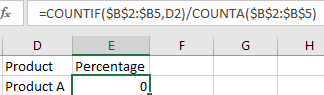In this case we can get the percentage by ‘Sales of each product/Total Sales’, so we can use a simple formula to calculate the percentage.

Step 1: In E2, enter the formula =B2/SUM(\$B\$2:\$B\$5).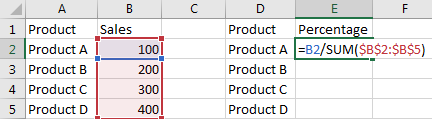Step 2: Click Enter to get the result.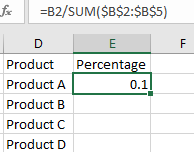Step 3: Drag the fill handle to fill the following cells. Verify that percentage is displayed for each product.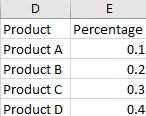## Method 3: Calculate the Percentage by Insert a PivotTable in Excel

See the table below. It is a little more complex than above two tables. Products are duplicated displayed in the list. To calculate the percentage for each product, we can use a PivotTable.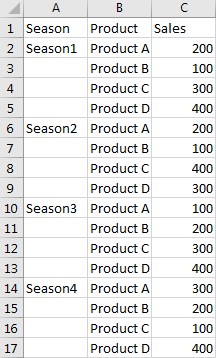Step 1: Click Insert->Recommended PivotTables.Step 2: In the pops up Create PivotTable, check on ‘Select a table or range’, click the arrow button and select the range you want to count the percentage on sheet1. In this case, you can select \$A\$1:\$C\$17. Then click OK.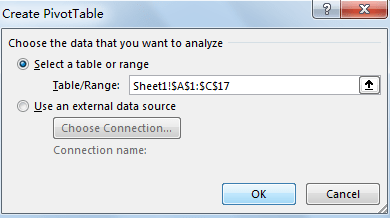Step 3: Verify that Recommended PivotTables window pops up. Click OK here.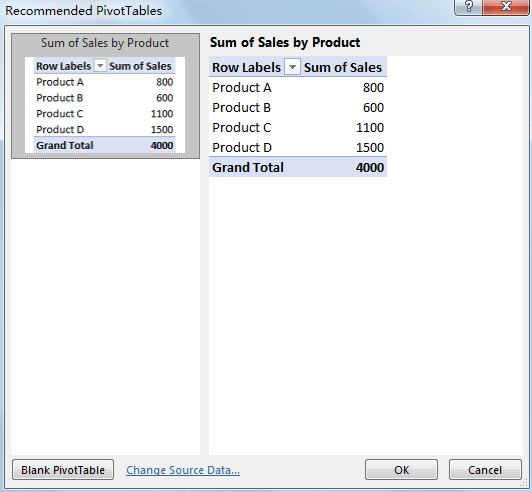Step 4: Verify that PivotTable is created on new sheet2.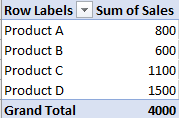Step 5: Double click on ‘Sum of Sales’ to load ‘Value Field Settings’.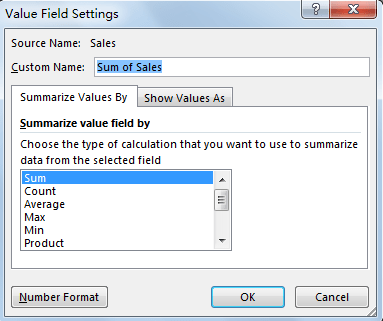Step 6: Click on ‘Show Values As’ tab. In ‘Show Values As’ dropdown list, click the arrow button, and select the third one ‘% of Column Total’.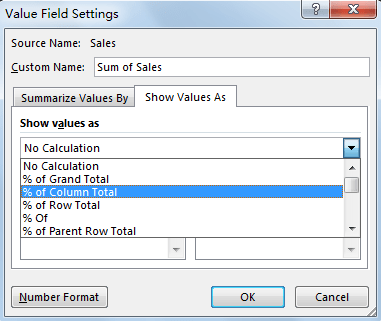Step 7: Update the Custom Name to ‘Percentage of Sales’, then click OK.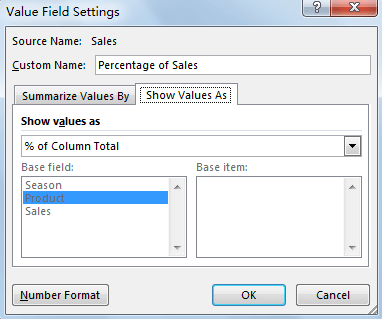Step 8: Verify that percentages are calculated properly for all products. You can also directly click on A3 to update the Row Labels per your requirement.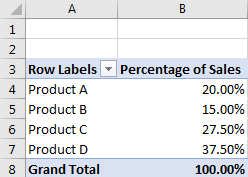### Related Functions

• Excel COUNTIF function
The Excel COUNTIF function will count the number of cells in a range that meet a given criteria. This function can be used to count the different kinds of cells with number, date, text values, blank, non-blanks, or containing specific characters.etc.= COUNTIF (range, criteria)…
• Excel COUNTA function
The Excel COUNTA function counts the number of cells that are not empty in a range. The syntax of the COUNTA function is as below:= COUNTA(value1, [value2],…)…